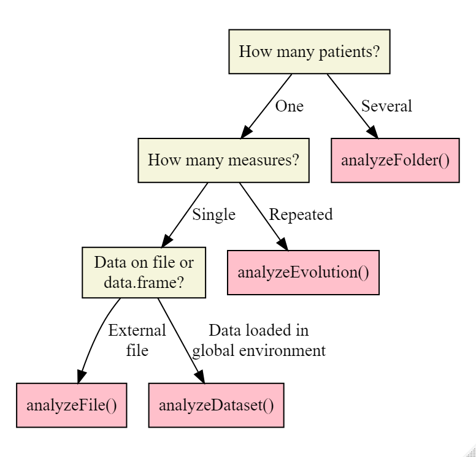# Workflow of the rPACI package

The rPACI package is developed to facilitate the calculation and interpretation of several indices for detecting corneal irregularities, and especially keratoconus. These indices were introduced in Ramos-López et al. (2011), Ramos-López et al. (2013) and Castro-Luna, Martínez-Finkelshtein, and Ramos-López (2020), and proved to be effective in detecting keratoconus and early keratoconus.

## Package installation

rPACI depends on the bnlearn package and uses functions from the ggplot2, tidyr and ggpubr packages as well. To install and load the package and its dependencies, open an R session and write the following code:

install.packages("rPACI", dependencies = TRUE)
library("rPACI")

## Available datasets

The rPACI package contains 11 datasets, real and simulated, with two different formats.

File name Source File format Directory Corneal condition
ds1.txt Real measurement rPACI system.file(“extdata”, package=“rPACI”) Normal
ds2.txt Simulated data rPACI system.file(“extdata”, package=“rPACI”) Normal
K01.txt Real measurement CSO system.file(“extdata”, package=“rPACI”) Keratoconus
K02.txt Real measurement CSO system.file(“extdata”, package=“rPACI”) Keratoconus
K03.txt Real measurement CSO system.file(“extdata”, package=“rPACI”) Keratoconus
N01.txt Real measurement CSO system.file(“extdata”, package=“rPACI”) Normal
N02.txt Real measurement CSO system.file(“extdata”, package=“rPACI”) Normal
S01.txt Real measurement CSO system.file(“extdata”, package=“rPACI”) Keratoconus suspect
simulated_2020_05_31.txt Simulated data rPACI system.file(“extdata/evolution/”, package=“rPACI”) Normal
simulated_2020_10_31.txt Simulated data rPACI system.file(“extdata/evolution/”, package=“rPACI”) Keratoconus suspect
simulated_2021_04_30.txt Simulated data rPACI system.file(“extdata/evolution/”, package=“rPACI”) Normal

The package includes three functions to read data: readCSO(), readrPACI() and readFile(). The two former ones read data in specific formats (explained below), while the latter one is a wrapper function able to read both formats. In general, we recommend using the more general wrapper function readFile() to read any file format.

To begin with, external files with a corneal topography in the format exported by some Placido disk topographers, especially those from CSO (a commercial brand), can be loaded using the function readCSO(). We recommend reading the Corneal topographers and data format vignette. This reading function allows to specify different parameters:

• filepath: The name of the file to be read.
• ringsTotal: The total (maximum) number of rings that may be available in the measurement (including incomplete rings or missing data; it depends on the particular device; by default 24).
• pointsPerRings: The number of points per ring that are digitized in the measurement (it depends on the particular device; by default 256).
• ringsToUse: The effective number of innermost rings to use (as long as they are complete if onlyCompleteRings = TRUE, otherwise it will be the actual number of complete rings; by default 15).
• onlyCompleteRings: A boolean value indicating whether to use only rings with complete data or not (by default, TRUE).
• NAvalues: A numerical value or vector indicating how NA values are codified in the file (by default c(-1, -1000)).

In the following example, a sample file with the corneal topography of a normal eye (included in the package as N01.txt) is used:

dataset1 = readCSO(filepath = system.file("extdata","N01.txt", package="rPACI"))

This function produces a data.frame in the usual format used by rPACI, i.e., a data frame with three columns (x and y coordinates of each point and its ring index) and a row per data point, according to the function parameters (by default, 24*256 = 6144 rows or data points).

head(dataset1)
#>           x           y ring index
#> 1 0.2022353 0.000000000          1
#> 2 0.2019057 0.004956506          1
#> 3 0.2014516 0.009896683          1
#> 4 0.2009069 0.014819783          1
#> 5 0.2003041 0.019728232          1
#> 6 0.1996709 0.024627055          1

On the other hand, external files with a corneal topography in the format exported by rPACI can also be loaded using the function readrPACI(). This function has two arguments: the path to the file (filepath) and the character used as column separator in the file (sep). In the following example, a sample file with the rPACI format (included in the package) is loaded:

dataset2 = readrPACI(filepath = system.file("extdata","ds1.txt", package="rPACI"))

This function returns a data.frame with the same characteristics as readCSO().

Finally, the general wrapper function readFile() can be used to read any of the aforementioned file formats. Internally, it determines the format of the specified file and applies either readCSO() or readrPACI() if possible, or else it throws an error (if none can be applied, which occurs when the file format does not fit any of these two available formats). This is the reading function recommended by default, as it is able to read any supported file format. The readFile() function takes the file path as a mandatory argument and, optionally, any of the arguments available for the other two reading functions. As a result, this function creates a data.frame in the usual format used by rPACI, as the other two functions do. In the following example, a sample file included in the package is loaded using the readFile() wrapper function:

dataset3 = readFile(filepath = system.file("extdata","N01.txt", package="rPACI"))

#>           x           y ring index
#> 1 0.2022353 0.000000000          1
#> 2 0.2019057 0.004956506          1
#> 3 0.2014516 0.009896683          1
#> 4 0.2009069 0.014819783          1
#> 5 0.2003041 0.019728232          1
#> 6 0.1996709 0.024627055          1

## Saving data

The function writerPACI() writes to disk a corneal topography dataset in the format used by rPACI. The file will be saved in structured plain text, which will possibly have a header, followed by a block of three separated columns, according to the usual format used by rPACI, i.e., a list with three columns (x and y coordinates of each point, and its ring index) and a row per data point.

If the given data.frame (named dataset) was produced using the function simulateData(), the resulting text file will also include the Parameters attribute (attr(dataset,'Parameters')) in its header, i.e., the list of parameters used for the simulation.

A file stored with writerPACI() can later be read using the general reader function readFile() or the specific reader function readrPACI().

The following piece of code shows how to use the writerPACI() function.

# Simulating an elliptic dataset, with ellipses axis ratio of 0.8 and an orientation of 45 degrees.
dataset = simulateData(rings = 18, pointsPerRing = 300, ellipticAxesRatio = 0.8, ellipticRotation = 45)

# Now the dataset can be saved to file using 'writerPACI' (check the working directory before saving):
writerPACI(dataset, "datasetFile.txt")

See more details about the file structure in the Corneal topographers and data format vignette and about simulation parameters in the Simulating corneal datasets vignette.

## Computing the Placido irregularity indices

When a corneal dataframe is available in the global environment, the Placido irregularity indices of this data set can be computed by calling function computePlacidoIndices(), which takes the object returned by readFile() (or readCSO() or readrPACI()) as its only argument:

results_N = computePlacidoIndices(dataset1)
results_N
#>        Diagnose          NBI         GLPI     PI_1     PI_2     PI_3       SL
#> 1 Normal cornea 2.409939e-07 1.647066e-09 13.21839 20.48343 17.69205 36.48707
#>       AR_1     AR_2     AR_3     AR_4     AR_5
#> 1 42.59781 21.72575 24.59397 21.37111 22.65632

The object returned by computePlacidoIndices() is of class data.frame and contains 12 columns and 1 row (because we are analyzing only one eye). The first column of the returned data.frame is the diagnosis, based on the GLPI index, which can be either Irregular cornea (GLPI $$\geq$$ 70), Suspect cornea (30 $$\leq$$ GLPI <70) or Normal cornea (GLPI <30). The next column is the Naive Bayes Index (NBI), which ranges between 0 and 100 and can be interpreted as the probability of suffering from keratoconus. The remaining columns correspond to the primary indices, defined in the Mathematical definition of the indices vignette.

## Plotting the results

The results of the previous analysis can be plotted using function plotSingleCornea(), which takes 3 arguments: dataset, a data.frame containing the corneal topography data, i.e., the object returned by readFile() (or readCSO() or readrPACI()); PlacidoIndices, a data.frame containing the computed Placido indices, i.e., the object returned by computePlacidoIndices(); and, optionally, filename, a character vector to be displayed on the plot (for instance, the filename of the corneal topography dataset).

  plotSingleCornea(dataset1, results_N, filename = "N01.txt")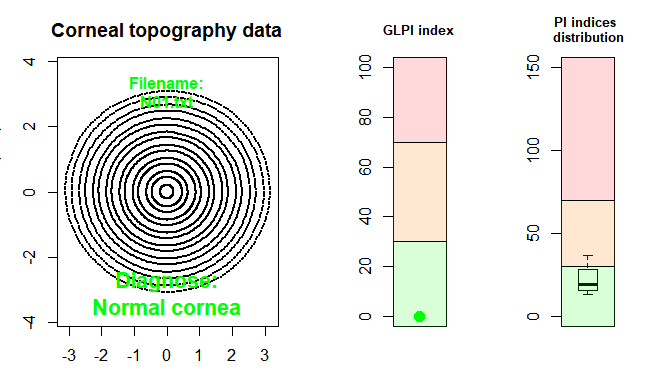The left-hand side of the figure shows the input data, whereas the right-hand side shows two charts: the GLPI index plot, which visually indicates the value taken by this index on a colored scale of possible values, and the PI indices distribution, which shows the distribution of the $$PI_1$$, $$PI_2$$, $$PI_3$$ and $$SL$$ indices in a boxplot placed on a scale of possible values. The colors of the charts indicate whether the indices fall within the normal cornea region (green color), suspicious cornea region (orange color), or irregular cornea region (red color). In the example shown, we can clearly see that the eye is diagnosed as normal.

## Using the wrapper function to analyze one external file

Alternatively to use the readFile(), computePlacidoIndices() and plotSingleCornea() functions, the wrapper function analyzeFile() can be used instead, which takes 2 arguments: path, which is a character string indicating the location of the corneal topography file, and drawplot, which is a logical argument indicating whether the results should be plotted or not. This function returns a data.frame containing the same information as the object returned by computePlacidoIndices() and, optionally, the plot returned by plotSingleCornea() if the argument drawplot is TRUE.

  res_K = analyzeFile(system.file("extdata","K01.txt", package="rPACI"), drawplot=TRUE)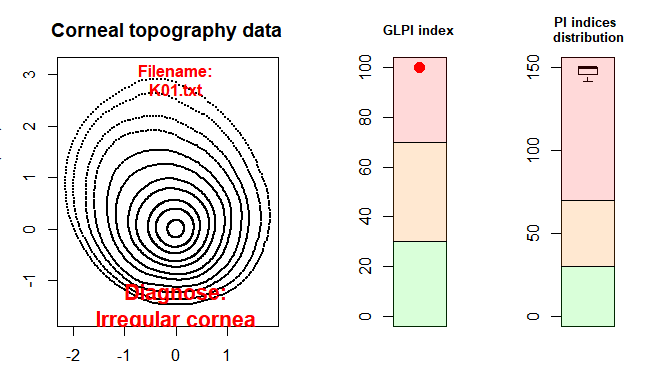res_K
#>           Diagnose NBI GLPI PI_1 PI_2 PI_3       SL     AR_1     AR_2     AR_3
#> 1 Irregular cornea 100  100  150  150  150 141.8956 118.9002 71.86885 82.74312
#>       AR_4     AR_5
#> 1 73.32595 76.20055

In this example, the results indicate that the patient’s cornea is irregular. In this case, the plot shows a GLPI index of 100 and a distribution of PI indices ranging from 100 to 130.

## Using the wrapper function to analyze one dataset

The function analyzeFile() reads a file stored in our computer. But, what if we already have a corneal data frame loaded in our global environment? We can use the wrapper function analyzeDataset() instead. This function is a wrapper of computePlacidoIndices() and plotSingleCornea(), so that it returns the same objects as analyzeFile(). This is a convenient function, for instance, when we use the simulateData() function (for more details on this function, see the Simulating corneal datasets vignette) or have previously loaded the data using the readFile() function.

analyzeDataset() takes two arguments: dataset, which is a corneal topography dataset in the format supported by rPACI; and drawplot, which is an optional logical parameter indicating whether a plot of results should be displayed or not (by default, TRUE).

# Generate a sample dataset
dataset = simulateData(rings = 12, maximumMireDisplacement = 0.2, mireDisplacementAngle = 50)

# Analyze the dataset
res_dataset = analyzeDataset(dataset = dataset)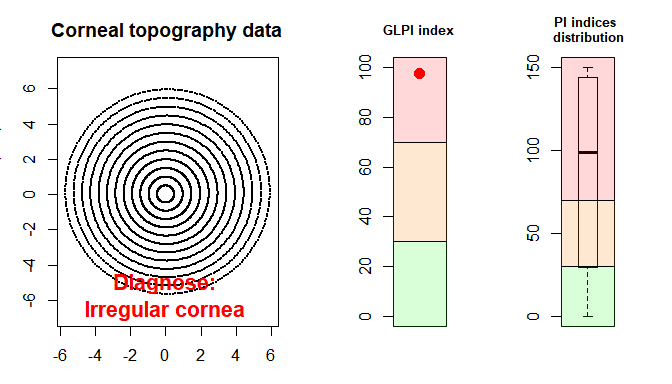res_dataset
#>           Diagnose NBI     GLPI PI_1    PI_2 PI_3       SL AR_1 AR_2 AR_3 AR_4
#> 1 Irregular cornea 100 97.68146  150 138.148    0 59.58768    0    0    0    0
#>   AR_5
#> 1    0

## Analyzing multiple patients simultaneously

So far, analyzeFile() and analyzeDataset() can only be used to analyze one dataset (i.e., one patient’s eye) at a time. In order to analyze multiple files simultaneously, the function analyzeFolder() can be used. This function takes 4 arguments: path, to indicate the location of the folder containing the files; fileExtension, which indicates the extension of the files, is set to ‘txt’ by default; individualPlots, which is an optional logical argument indicating whether the plot for each file should be displayed or not; and summaryPlot, which is an optional logical argument indicating whether a summary plot of all files analyzed should be displayed or not. If the argument summaryPlot() is TRUE, then a barplot showing the absolute frequency of each possible value of diagnosis is depicted.

resultsAll = analyzeFolder(path = system.file("extdata", package="rPACI"), individualPlots = T, summaryPlot = T)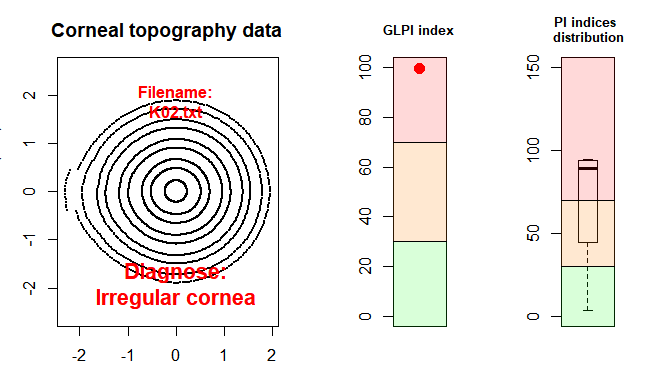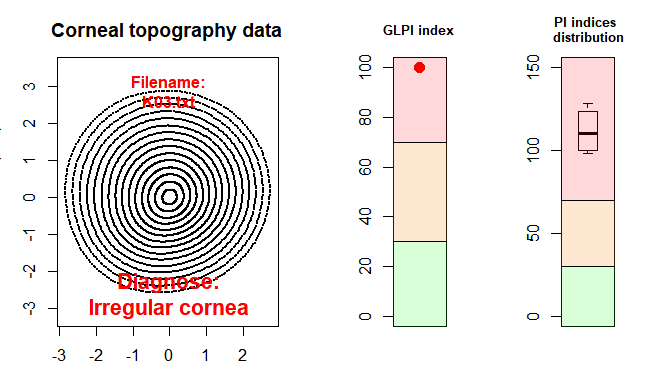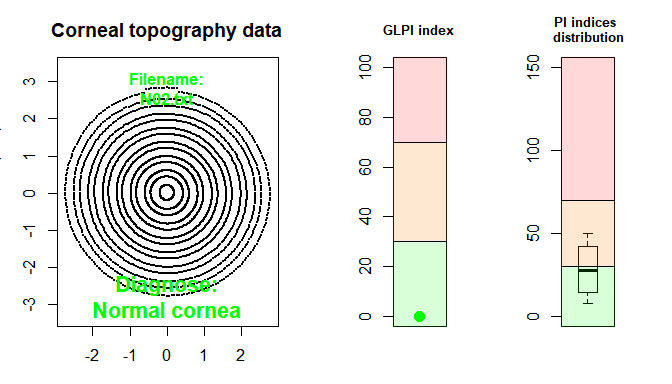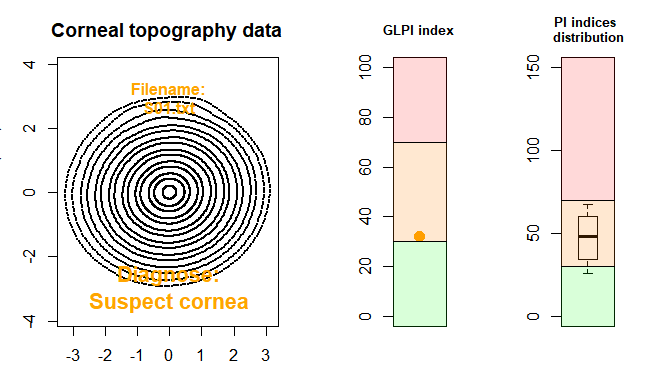#> Warning in computePlacidoIndices(data): Too many rings: using only the 15
#> innermost rings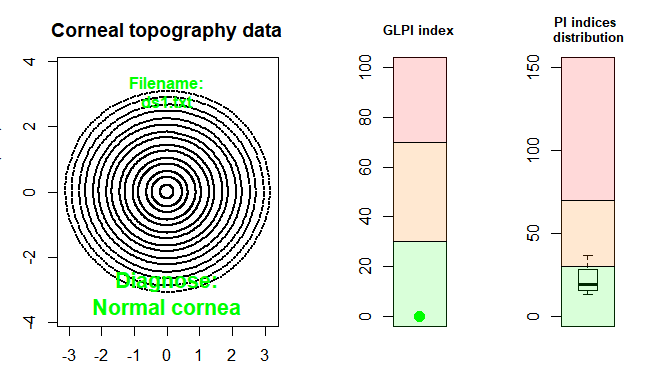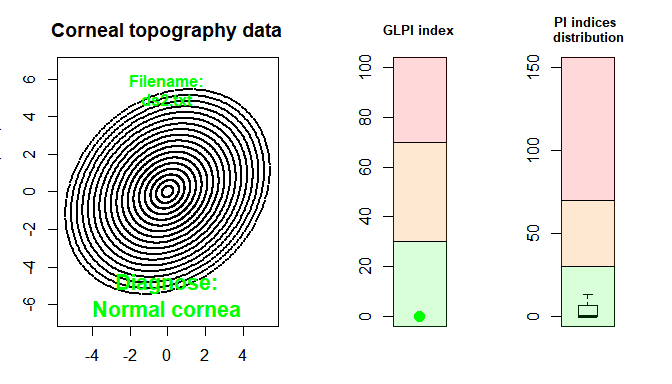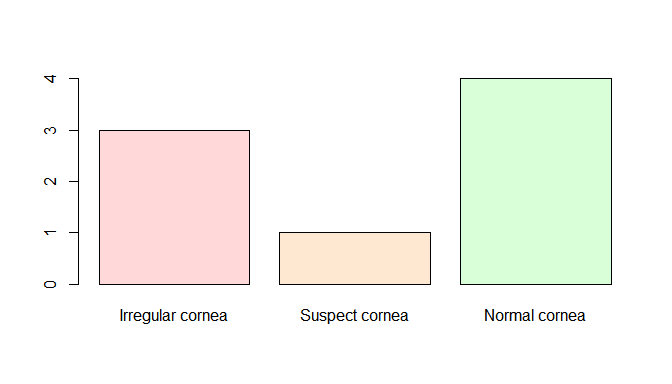resultsAll
#>           Diagnose          NBI         GLPI       PI_1      PI_2      PI_3
#> 1 Irregular cornea 1.000000e+02 1.000000e+02 150.000000 150.00000 150.00000
#> 3 Irregular cornea 1.000000e+02 1.000000e+02 128.208980 101.31179 118.75232
#> 2 Irregular cornea 1.000000e+02 9.966268e+01  94.466174  85.07159  93.06011
#> 6   Suspect cornea 1.012263e-05 3.206200e+01  42.986774  52.80403  67.65683
#> 5    Normal cornea 1.079840e-05 5.498169e-03   8.053099  20.75008  49.72166
#> 4    Normal cornea 2.362192e-07 1.647066e-09  13.218393  20.48343  17.69205
#> 7    Normal cornea 2.295121e-07 1.647066e-09  13.218393  20.48343  17.69205
#> 8    Normal cornea 7.446675e-04 0.000000e+00   0.000000   0.00000   0.00000
#>           SL      AR_1     AR_2     AR_3     AR_4     AR_5 Filename
#> 1 141.895574 118.90021 71.86885 82.74312 73.32595 76.20055  K01.txt
#> 3  98.410454  56.83052 56.07982 47.44765 58.58303 51.29579  K03.txt
#> 2   3.721893   0.00000  0.00000  0.00000  0.00000  0.00000  K02.txt
#> 6  26.079816  39.21140 25.91339 30.93766 27.35318 29.50081  S01.txt
#> 5  34.660291  57.19127 32.79401 37.87577 33.82410 36.36971  N02.txt
#> 4  36.487065  42.59781 21.72575 24.59397 21.37111 22.65632  N01.txt
#> 7  36.487065  42.59781 21.72575 24.59397 21.37111 22.65632  ds1.txt
#> 8  13.230459   0.00000  0.00000  0.00000  0.00000  0.00000  ds2.txt

This function returns a data.frame containing 13 columns and as many rows as files analyzed. The first 12 columns correspond to the diagnosis and the indices, as in the object returned by computePlacidoIndices(). The last column corresponds to the file name so that a specific patient can be easily found. To see the diagnosis for each analyzed file, the first and last columns can be selected:

resultsAll[,c(13,1)]
#>   Filename         Diagnose
#> 1  K01.txt Irregular cornea
#> 3  K03.txt Irregular cornea
#> 2  K02.txt Irregular cornea
#> 6  S01.txt   Suspect cornea
#> 5  N02.txt    Normal cornea
#> 4  N01.txt    Normal cornea
#> 7  ds1.txt    Normal cornea
#> 8  ds2.txt    Normal cornea

Note that the rows are sorted from Irregular to Normal cornea. In this example, three eyes are diagnosed as irregular, one as suspect, and four as normal. This is an easy and straightforward way to check if any patient potentially suffers from keratoconus.

## Analyzing one patient over time

In order to examine the evolution of a patient’s eye over time, the function analyzeEvolution() can be used. This function takes two arguments: data, which can be either 1) the path of a folder that contains corneal topography files, with format supported by rPACI, or 2) a list containing properly formatted data (loaded from a file using the function readFile() (or readCSO() or readrPACI()), simulated using simulateData(), or by other ways, as long as it meets the dataset requirements). If data is a path to a folder, the second argument, fileExtension, must be specified and all the files (with the given extension) in that folder will be assumed to be corneal topography files of a patient’s eye and, therefore, will be loaded.

Moreover, it will be assumed that the temporal arrangement is the alphabetical order of the filenames. Therefore, it is advised to use a proper file name, for instance using this date format: ‘YYYY-MM-DD.txt’. On the other hand, if the data are stored in a list, it will be assumed that the temporal order corresponds with the index of each dataset in the list.

The next example simulates three patient’s measurements over time using the function simulateData() and then analyzes these files:

# Simulate the patient's measures over time
dataT1 = simulateData(rings = 12, maximumMireDisplacement = 0.15, mireDisplacementAngle = 10)
dataT2 = simulateData(rings = 12, maximumMireDisplacement = 0.15, mireDisplacementAngle = 45)
dataT3 = simulateData(rings = 12, maximumMireDisplacement = 0.2, mireDisplacementAngle = 50)

# Create a list containing the data
data = list(
dataT1 = dataT1,
dataT2 = dataT2,
dataT3 = dataT3
)

# Analyze the data over time
analyzeEvolution(data = data)

This other example imports the corneal data from disk and analyzes the files:

# Specify a folder path to analyze a patient's evolution over time
analyzeEvolution(data = system.file("extdata/evolution/", package="rPACI"), fileExtension = 'txt')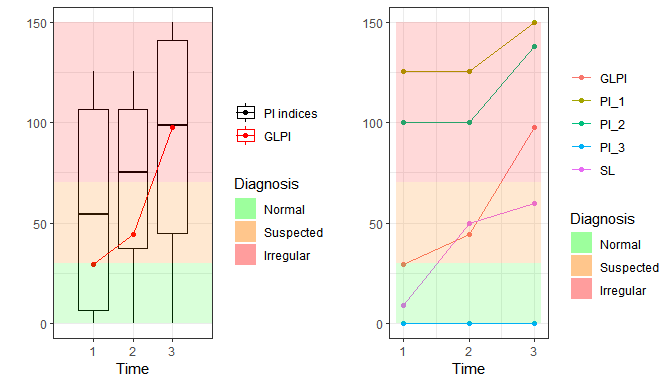#>           Diagnose NBI     GLPI     PI_1      PI_2 PI_3        SL AR_1 AR_2
#> 1    Normal cornea 100 29.21194 125.7446  99.99175    0  8.816349    0    0
#> 2   Suspect cornea 100 44.11881 125.7446  99.99174    0 50.000000    0    0
#> 3 Irregular cornea 100 97.68146 150.0000 138.14797    0 59.587680    0    0
#>   AR_3 AR_4 AR_5 Time
#> 1    0    0    0    1
#> 2    0    0    0    2
#> 3    0    0    0    3

The analyzeEvolution() function returns a data.frame containing 13 columns and as many rows as files analyzed. The first 12 columns correspond to the diagnosis and the indices, as in the object returned by computePlacidoIndices(). The last column corresponds to the time step at which the measures were taken. Moreover, two temporal plots are returned. The left-hand side plot shows the $$GLPI$$ index, represented by a red line, and the boxplots of the primary indices $$PI_1$$, $$PI_2$$, $$PI_3$$, and $$SL$$ over time. The right-hand side plot shows the times series of these five indices, $$GLPI$$, $$PI_1$$, $$PI_2$$, $$PI_3$$, and $$SL$$, individually. Finally, both plots present a colored background, corresponding with the final diagnosis of the patient.

In this example, the results indicate that the patient’s cornea was normal at time 1, suspected at time 2, and irregular at time 3.

## Summary

To summarize, the following description chart can help decide which function to use depending on the specific case to analyze.